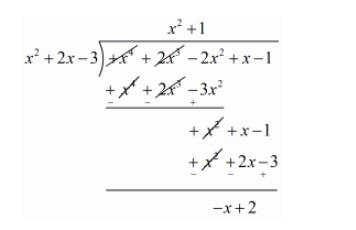# What must be added to the polynomial f(x) = x4 + 2x3 − 2x2 + x − 1 so

Question:

What must be added to the polynomial $f(x)=x^{4}+2 x^{3}-2 x^{2}+x-1$ so that the resulting polynomial is exactly divisible by $x^{2}+2 x-3 ?$

Solution:

We know that,

$f(x)=g(x) \times q(x)+r(x)$

$f(x)-r(x)=g(x) \times q(x)$

$f(x)+\{-r(x)\}=g(x) \times q(x)$

Clearly, Right hand side is divisible by $g(x)$.

Therefore, Left hand side is also divisible by $g(x)$. Thus, if we add $-r(x)$ to $f(x)$, then the resulting polynomial is divisible by $g(x)$.

Let us now find the remainder when $f(x)$ is divided by $g(x)$.Hence, we should add $-r(x)=x-2$ to $f(x)$ so that the resulting polynomial is divisible by $g(x)$.